﻿ 基于分布估计算法的战能转化控制方法
«上一篇文章快速检索 高级检索

 哈尔滨工程大学学报2018, Vol. 39Issue (12): 2046-2053  DOI: 10.11990/jheu.2018020040

### 引用本文ZHANG Dongjun, LI Xiao, WU Hong, et al. Combat energy transform control method based on distribution estimation algorithm[J]. Journal of Harbin Engineering University, 2018, 39(12), 2046-2053. DOI: 10.11990/jheu.201802004.### 文章历史

1. 92337部队, 辽宁 大连 116023;
2. 96714部队, 福建 永安 366000

Combat energy transform control method based on distribution estimation algorithm
ZHANG Dongjun 1, LI Xiao 1, WU Hong 1,2, WANG Shi 1
1. 92337 Troops, Dalian 116023, China;
2. 96714 Troops, Yong'an 366000, China
Abstract: The traditional operational experiment method of combat process does not explore the law of war based on the dynamic game characteristics of war; thus, it is difficult to effectively uncover the fog of war and obtain the winning mechanism. Considering this problem, a combat energy control method for operational experiment is proposed in this paper. First, to formally define dynamic capability, combat behavior, equipment systems, and combat energy are introduced, and the relationship between them is established. The combat energy comprises seven factors:detection, concealment, defense, attack, accusation, communication, and security. Second, considering combat energy control, a combat energy loop of amassment-perception-delivery-transformation, which effectively interprets the phase, progress, objective, and parallelism of operational activity, is built; the combat transform coefficient, which is composed of effect, peak, time, phase, density, and slope, is designed to identify combat energy. Third, the distribution estimation algorithm is introduced in the combat energy optimization control; the optimization phase is divided, and the behavior coding is designed according to the combat energy loop. The individual production mode is designed based on the combat transform coefficient. Experiments show that the proposed method is superior to the traditional method in terms of optimization efficiency and effect, and it can promote operational experimentation more effectively, providing a quantitative analysis method for research of combat law training methods.
Keywords: operational experiment    combat energy    combat potential    combat energy loop    combat energy transform    distribution estimation algorithm    combat behavior    dynamic game    optimization

1 面向作战实验的动态能力表达 1.1 作战行为

 $C = \left\{ {{C_1},{C_2}, \cdots ,{C_{{n_C}}}} \right\}$ (1)

 $O = \left\{ {{O_1},{O_2}, \cdots ,{O_{{n_O}}}} \right\}$ (2)

${O_i} \triangleleft C = {\mathit{\Omega }_{{O_i} \triangleleft C}} = \left\{ {{C_1}, {C_2}, \cdots , {C_{{n_{{O_i} \triangleleft C}}}}} \right\}$，表示能产生操纵行为Oi的指挥行为集合${\mathit{\Omega }_{{O_i} \triangleleft C}}$

${C_i} \triangleright O = {\mathit{\Omega }_{{C_i} \triangleright O}} = \left\{ {{O_1}, {O_2}, \cdots , {O_{{n_{{C_i} , O}}}}} \right\}$，表示指挥行为Ci下的操纵行为集合${\mathit{\Omega }_{{C_i} \triangleright O}}$

1.2 装备系统

 $S = \left\{ {{S_1},{S_2}, \cdots ,{S_{{n_S}}}} \right\}$ (3)

 $M = < T,X,S,Y,{\delta _{{\rm{ext}}}},{\delta _{{\rm{int}}}},\lambda ,\tau >$ (4)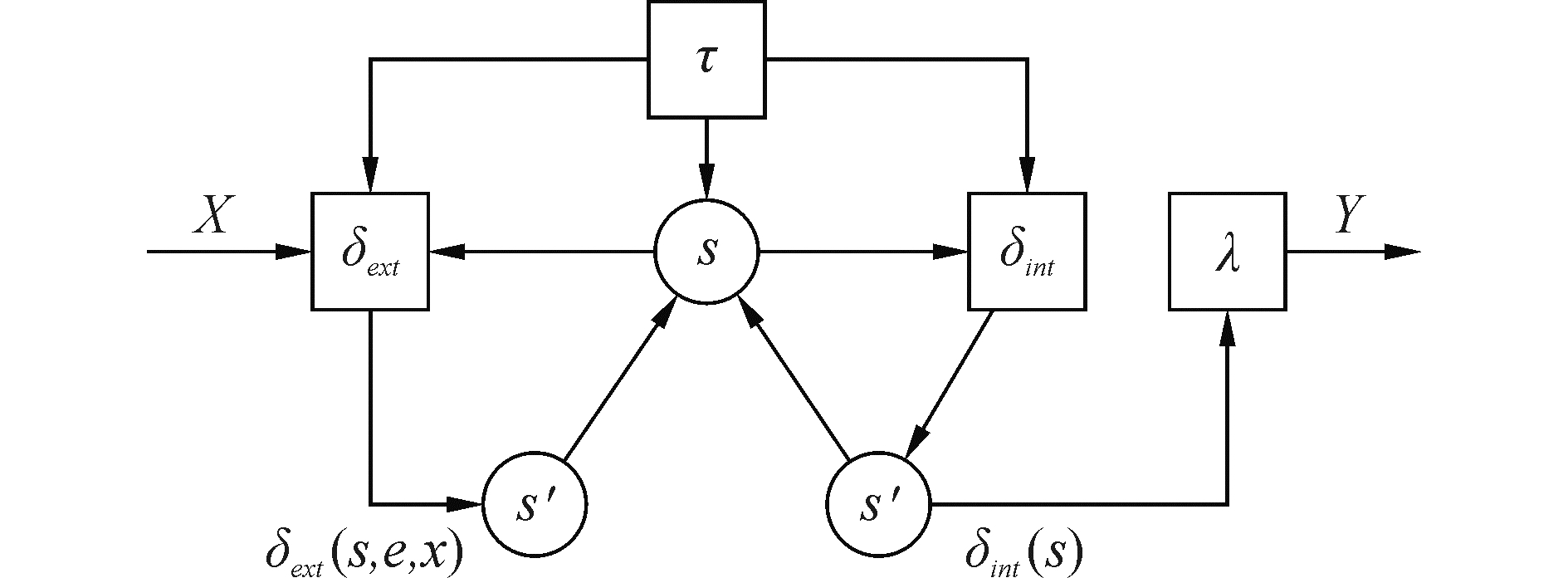Download: 图 1 离散事件系统规范 Fig. 1 Discrete event system specification

${S_i} \triangleleft O = {\mathit{\Omega }_{{S_i} \triangleleft O}} = \left\{ {{O_1}, {O_2}, \cdots , {O_{{n_{{S_i} \triangleleft O}}}}} \right\}$，表示能改变装备系统Si状态的操纵行为集合${\mathit{\Omega }_{{S_i} \triangleleft O}}$

${O_i} \triangleright S = {\mathit{\Omega }_{{O_i} \triangleright s}} = \left\{ {{S_1}, {S_2}, \cdots , {S_{{n_{{O_i} , S}}}}} \right\}$，表示操纵行为Oi造成状态变化的装备系统集合${\mathit{\Omega }_{{O_i} \triangleright s}}$

1.3 作战能量

 $E = \left( {{E_{Dt}},{E_E},{E_{Df}},{E_A},{E_C},{E_I},{E_G}} \right)$ (5)

${E_i} \triangleleft S = {\mathit{\Omega }_{{E_i} \triangleleft s}} = \left\{ {{S_1}, {S_2}, \cdots , {S_{{n_{{E_i} \triangleleft S}}}}} \right\}$，表示能对作战能量Ei产生影响的装备系统集合${\mathit{\Omega }_{{E_i} \triangleleft s}}$

${S_i} \triangleright E = {\mathit{\Omega }_{{S_i} \triangleright E}} = \left\{ {{E_1}, {E_2}, \cdots , {E_{{n_{{S_i} \triangleright E}}}}} \right\}$，表示装备系统Si能够产生影响的作战能量集合${\mathit{\Omega }_{{S_i} \triangleright E}}$

2 战能环及战能转化系数 2.1 战能环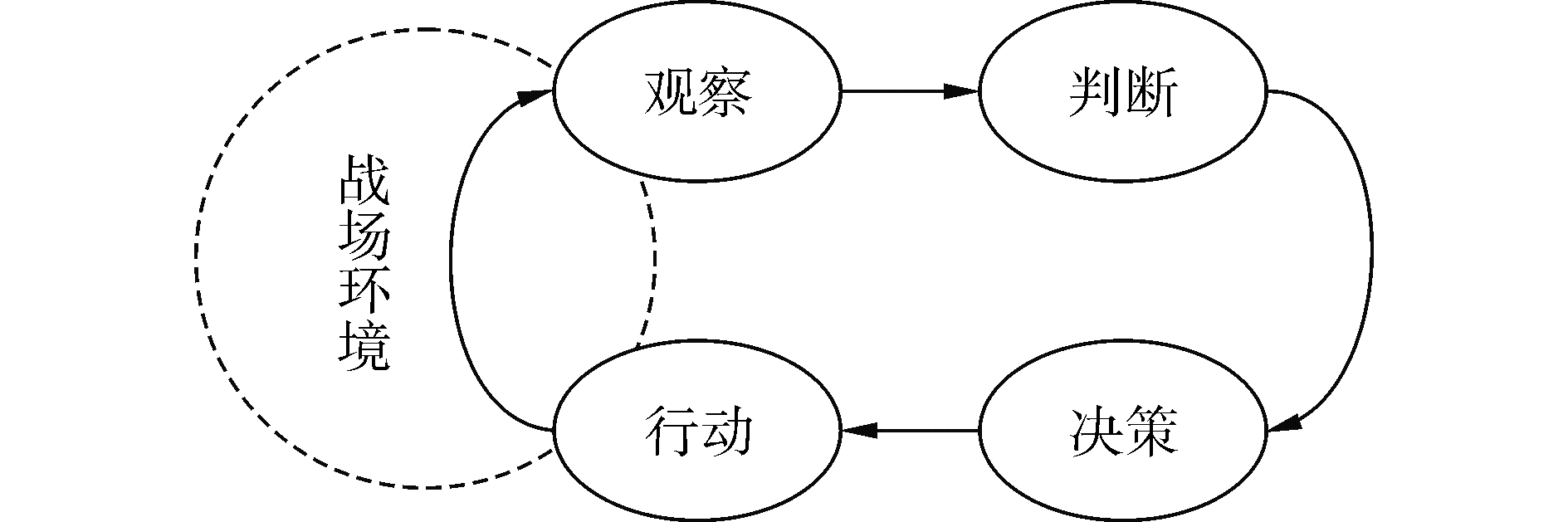Download: 图 2 OODA环 Fig. 2 OODA loop

1) 观察，利用各类侦察探测装备对战场环境进行观察和感知，从战场环境中搜集目标的位置、状态、属性等信息；

2) 判断，将观察环节收集到的信息和数据进行处理和融合，并对当前的战场态势进行评估；

3) 决策，依据战场态势制定行动计划和方案；

4) 行动，依据计划方案或上级命令指示实施相应行动。

OODA环具有周期性和嵌套性，周期性意味着一个环结束时，另一个新环的开始，周期性的大小与作战兵力的规模、空间范围、作战样式有关；嵌套性意味着环与环以嵌套的形式关联。目前，OODA被广泛应用于作战问题分析中，它可以很好地表述作战行动的阶段性、递进性，但无法有效诠释作战行动的目的性、并行性。如搜攻任务中，在抵达作战区域时，可为搜索、打击、防御进行提前布势，在搜索阶段同样可为打击、防御进行提前布势等。鉴于此，研究提出战能积蓄-感知-传递-转化的战能环，如图 3所示，其中：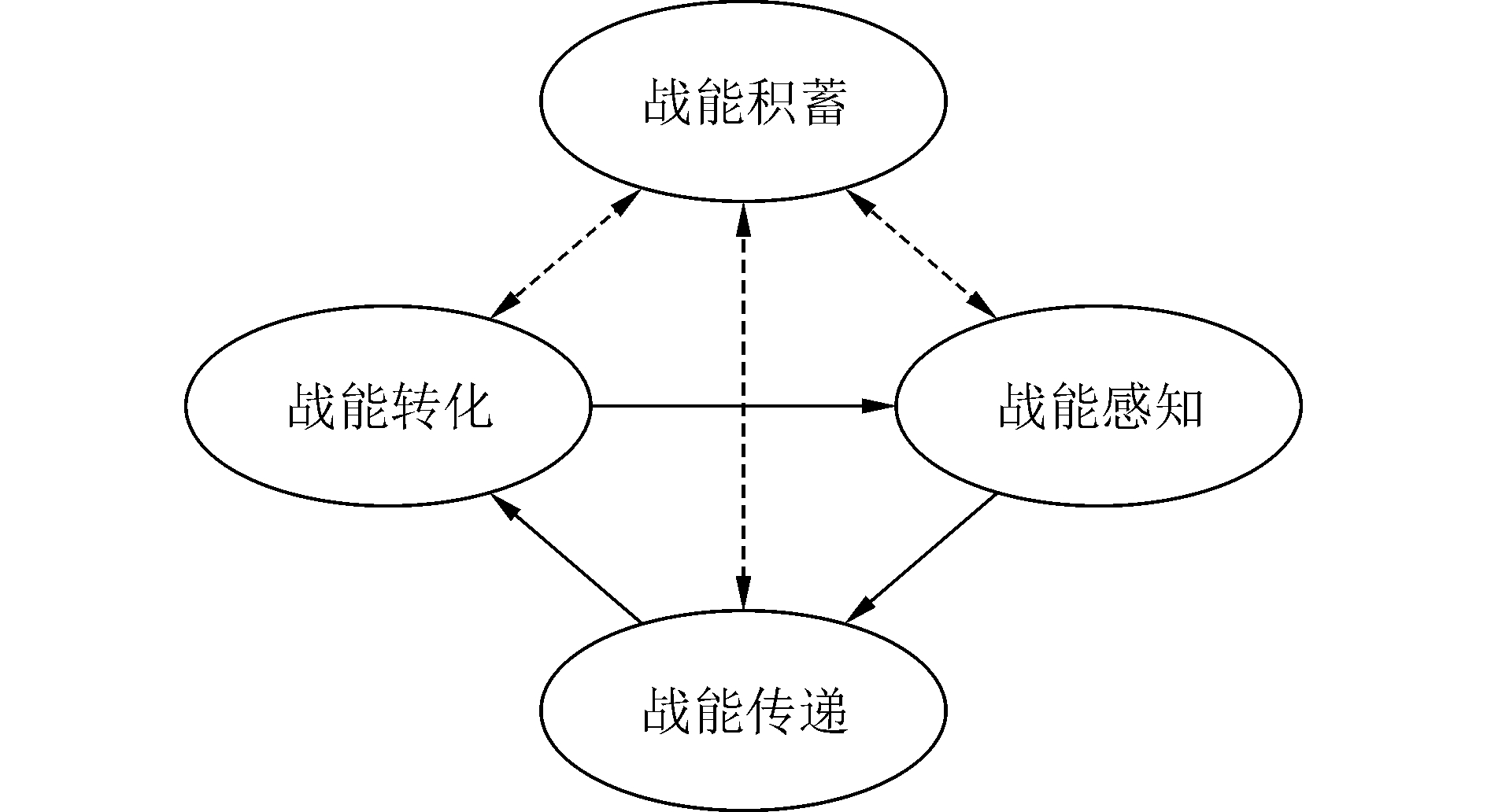Download: 图 3 战能环 Fig. 3 Combat energy loop

1) 战能积蓄(EA)，在作战进程中，以主行动目标为约束条件，基于作战行为的并行性，开展战能感知、传递和转化的优化布势。

2) 战能感知(EP)，在行为驱动下，充分考虑自身隐蔽情况，基于传感器获取敌方目标信息，主要涉及探测战能、隐蔽战能、保障战能。

3) 战能传递(ED)，在行为驱动下，完成目标分配及方案下发，以实现战能感知到战能转化的变迁，主要涉及指控战能、通信战能、保障战能。

4) 战能转化(ET)，在行为驱动下，基于攻击武器和防御武器，对敌实施攻击或防御，主要涉及攻击战能、防御战能、保障战能。

2.2 战能转化系数

 $\eta = \left( {{\eta _1},{\eta _2}, \cdots ,{\eta _{{n_\eta }}}} \right) = f\left( {E,{\rm{EP}},{\rm{ED}},A} \right)$ (6)

3 基于分布估计算法的战能转化控制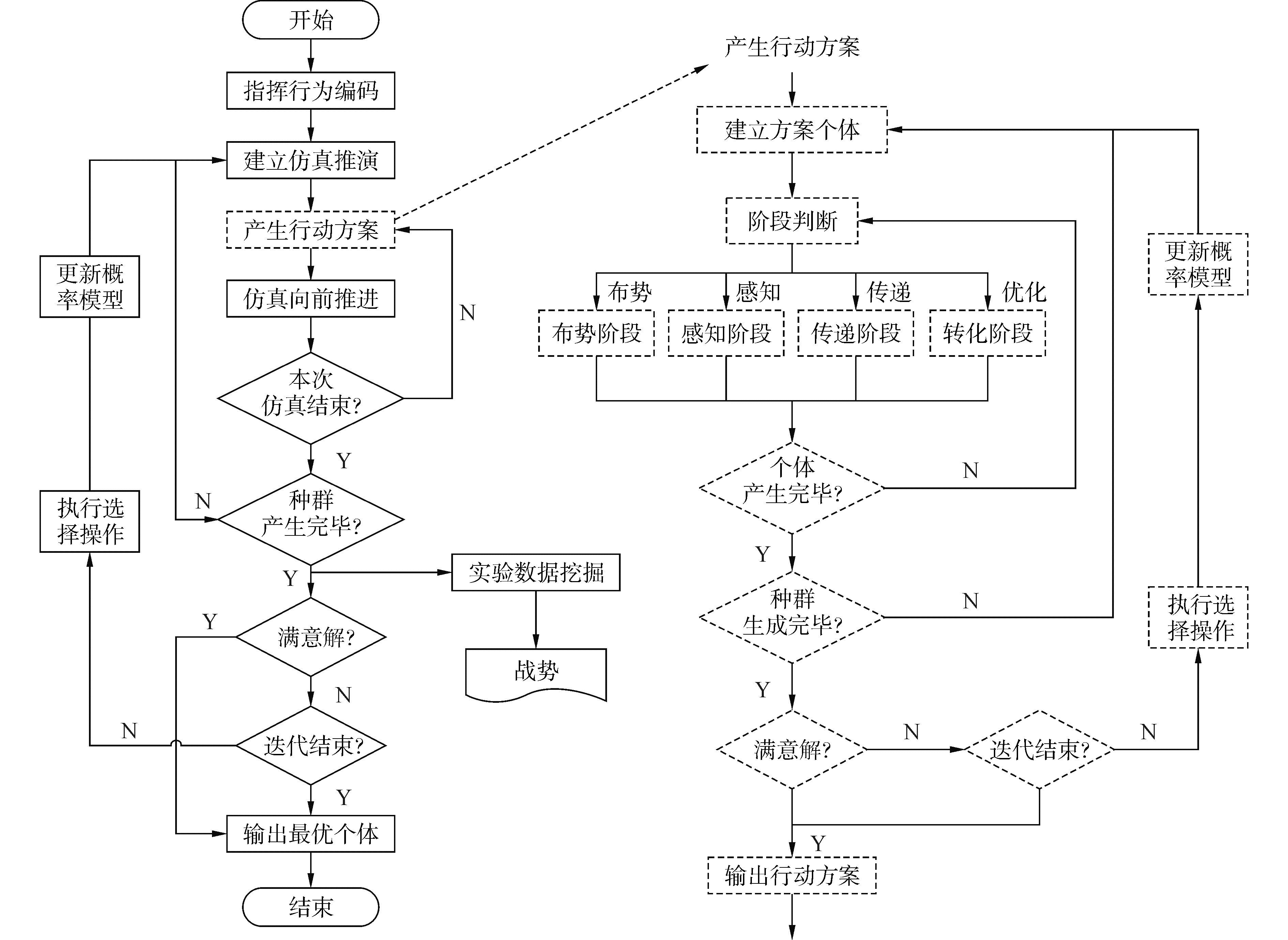Download: 图 4 基于战能环的优化框架 Fig. 4 Optimize frame based on combat energy loop
3.1 指挥行为编码

 $\left\{ \begin{array}{l} {\mathit{\Omega }_{{\rm{EP}} \triangleright C}} = \left\{ {{C_1},{C_2}, \cdots {C_{{n_{{\rm{EP}} \triangleright C}}}}} \right\}\\ {\mathit{\Omega }_{{\rm{ED}} \triangleright C}} = \left\{ {{C_1},{C_2}, \cdots {C_{{n_{{\rm{ED}} \triangleright C}}}}} \right\}\\ {\mathit{\Omega }_{{\rm{ET}} \triangleright C}} = \left\{ {{C_1},{C_2}, \cdots {C_{{n_{{\rm{ET}} \triangleright C}}}}} \right\} \end{array} \right.$ (7)

 ${P_{0,i,j}} = 1/{N_i}$ (8)

3.2 行动方案生成

1)在准备阶段，对感知、传递、转化中具有相对独立性的战能分量进行布势，具体步骤为：

 ${\rho _{i,j}} = {\eta _{l,j}}/\sum\limits_{l = 1}^{{N_i}} {{\eta _{l,j}}}$ (9)

 $\left\{ \begin{array}{l} {\rho _{i,j}} = {e_j}/\sum\limits_{j = 1}^{{N_i}} {{e_j}} \\ {e_{k,j}} = \sum\limits_{k = 1}^7 {{e_{k,j}}} /7 \end{array} \right.$ (10)

 ${P_{k,i,j}}^T = {\sigma _1}{P_{k - 1,i,j}} + \left( {1 - {\sigma _1}} \right){\rho _{i,j}}$ (11)

2) 在感知阶段，以感知为主目标，进行指挥行为序列化，具体操作步骤为：

 $\left\{ \begin{array}{l} {\rho _{i,j}} = {e_j}/\sum\limits_{j = 1}^{{N_i}} {{e_j}} \\ {e_{k,j}} = \sum\limits_{k = 1}^7 {{\omega _k}{e_{k,j}}} /7 \end{array} \right.$ (12)

3) 在传递阶段，以传递为主目标，进行指挥行为序列化，具体操作步骤与2)类似。

4) 在转化阶段，以转化为主目标，进行指挥行为序列化，具体操作步骤与2)类似，不同之处在于其概率分布的确定顺序为转化效果、转化时间、转化幅值、转化相位、转化斜率、转化密度。

3.3 精英种群选择

3.4 概率模型更新

 $\left\{ \begin{array}{l} {P_{k,i,j}} = {\sigma _2}{P_{k - 1,i,j}} + \left( {1 - {\sigma _2}} \right){{P'}_{k,i,j}}\\ {{P'}_{k,i,j}} = {N_{i,j}}/{N_i} \end{array} \right.$ (13)

3.5 实验数据挖掘

 $y = {\beta _0} + \sum\limits_{i = 1}^k {{\beta _i}{x_i}} + \sum\limits_{i = 1}^k {{\beta _{i,i}}x_i^2} + \sum\limits_i {\sum\limits_j {{\beta _{i,j}}{x_i}{x_j}} } ,\left( {i \ne j} \right)$ (14)

4 实例分析 4.1 实验设置

4.2 优化控制 4.2.1 战能设置

 $e = {\omega _A}{e_A} + {\omega _B}{e_B}$ (14)

4.2.2 战势设置

 ${\eta _1} = {\eta _{1,A}} + {\eta _{1,B}}$ (15)

4.2.3 优化设置

4.2.4 行为编码

 $C = \left\{ {{C_1},{C_2}, \cdots ,{C_{{n_C}}}} \right\},{n_C} = 17$

 $\begin{array}{l} {C_1} \to {C_i},{C_i} \to {C_1},{C_3} \to {C_4},{C_4} \to {C_5},\\ {C_5} \to {C_6},{C_6} \to {C_7},{C_8} \to {C_9},{C_9} \to {C_{10}},\\ {C_{10}} \to {C_{11}},{C_6} \to {C_7},{C_3} \triangleright {C_4},{C_4} \triangleright {C_5},\\ {C_5} \triangleright {C_6},{C_5} \triangleright {C_7},{C_8} \triangleright {C_9},{C_9} \triangleright {C_{10}},\\ {C_{10}} \triangleright {C_{11}} \end{array}$

4.2.5 实验结果

1) 转化效果演化进程。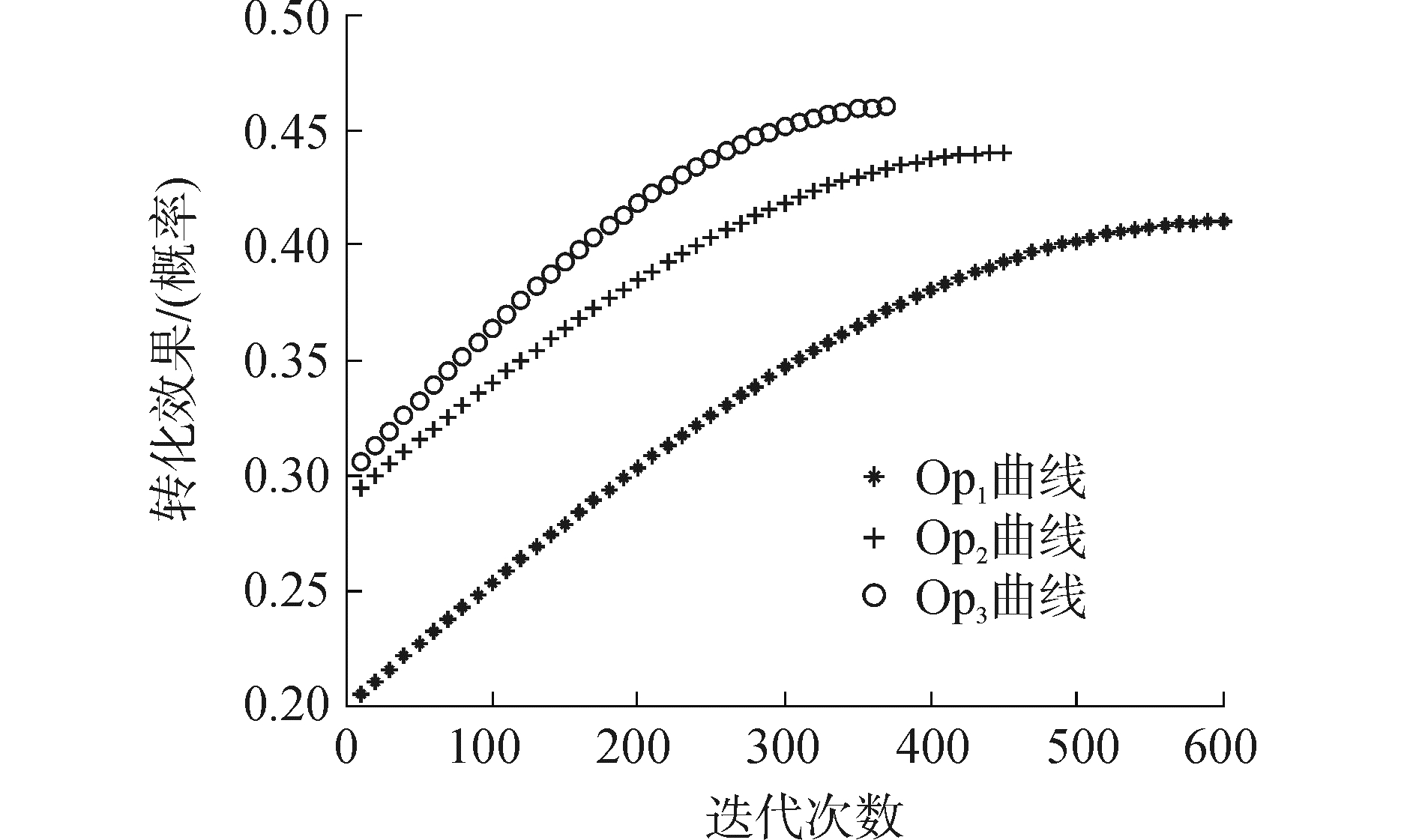Download: 图 5 转化效果演化进程 Fig. 5 Evolution process of transform effect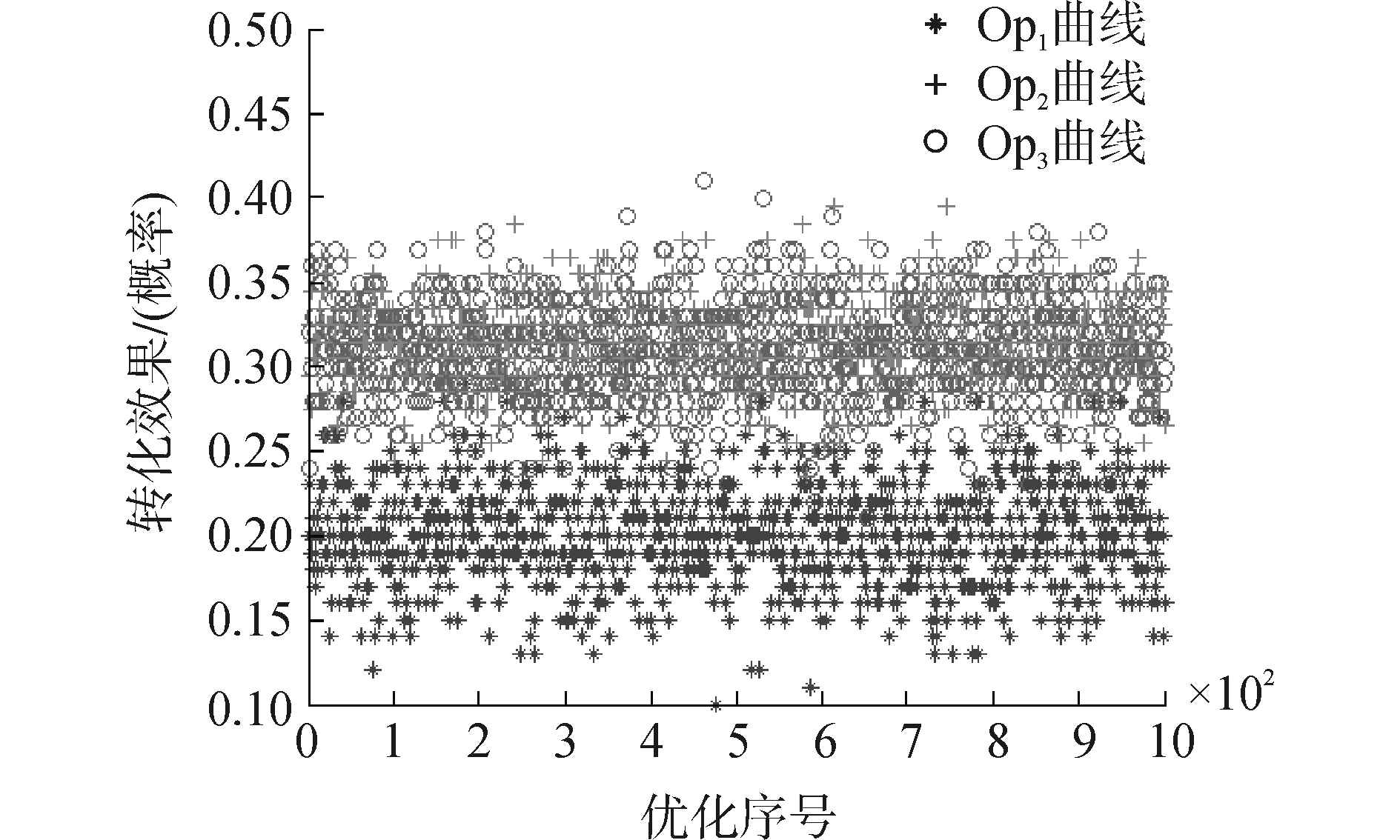Download: 图 6 初次迭代转化效果 Fig. 6 Transform effect of initial iteration

2) 优化效果均值方差。

1 000次优化的结果如图 7所示，构建方式为极坐标系：以均值为中心，以距离为极轴，以优化序号标识夹角；统计值如表 1所示。由图及统计值可知，Op3无论在优化质量还是优化效率方面，都要优于Op1、Op2Download: 图 7 转化效果分布 Fig. 7 Distribution of transform effects表 1 优化效果均值方差 Table 1 Mean value and variance of optimize result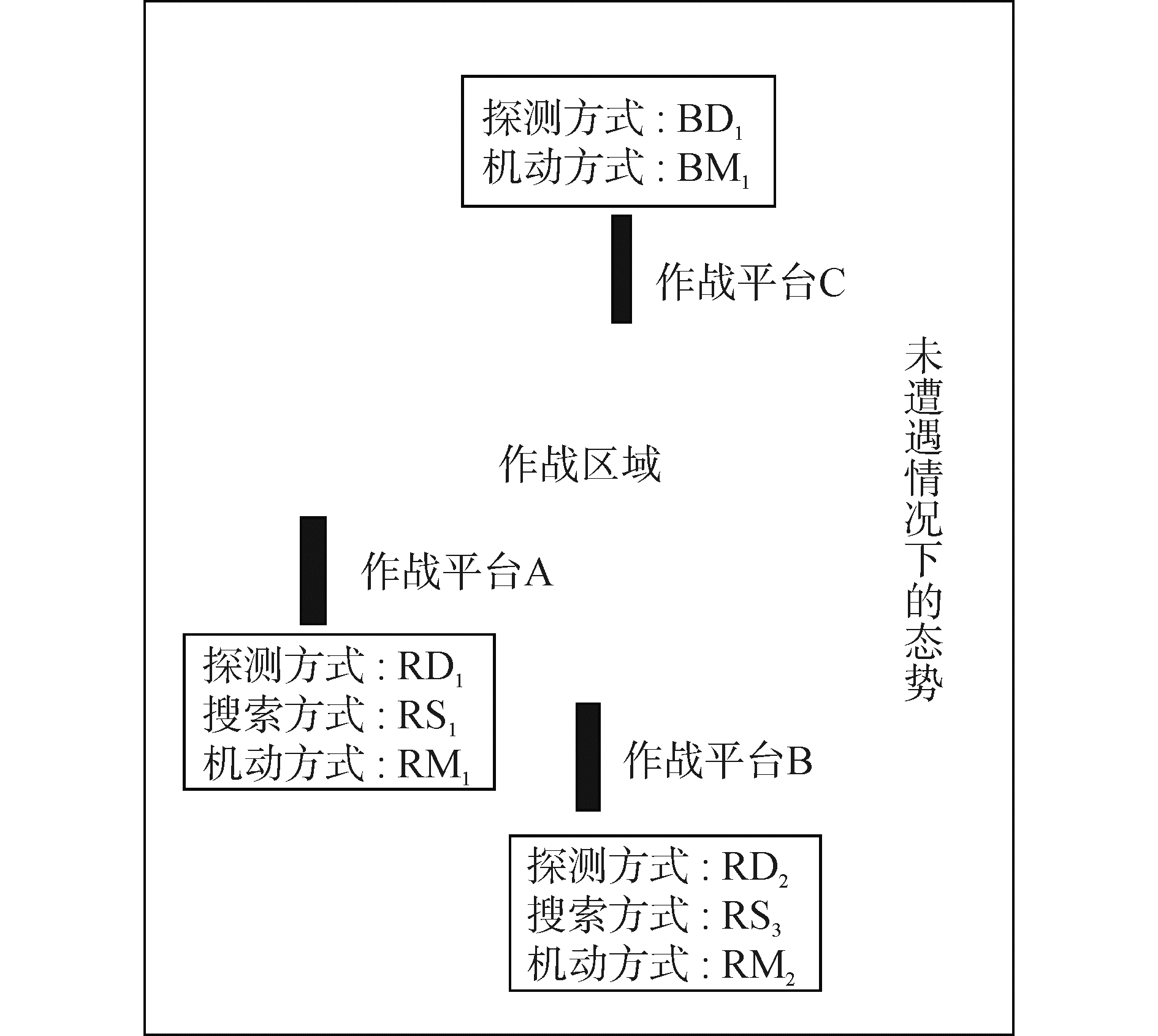Download: 图 8 红方搜攻任务方式 Fig. 8 Red side search and attack mode
5 结论

1) 借鉴AlphaGo的思路，将优化算法嵌入到作战进程中，以动态能力作为评价准则，通过红蓝双方智慧博弈来探究作战行为与装备系统的最佳组合；相比于传统方法，能取得更好的优化效率及效果，为研究战争规律提出了一种新的解题思路。

2) 辅助决策存在于部分作战系统中，但通常都属于基于规则库或解析模型的单步决策，即单点最优性；本研究为考虑多时段的博弈决策，具有全局最优性。因此，本研究不仅可以有效服务于战法训法创新；还可为辅助决策系统或智能指控系统的构建提供借鉴，或达到一定成熟度时，作为一型装备装配到作战平台上。

3) 作战大致可分为战略、战役、战术三个层次，本文的立足点为战术博弈，其能力状态的量化分析相对简单；如何量化战役、战略层红蓝双方的动态能力，并据此开展优化分析，以为高层指挥员进行兵力运用提供支持，是下一步研究的方向。

  李涛, 余军, 吴小勇, 等. 作战实验在海军装备体系建设中的应用研究[J]. 国防科技, 2014, 35(4): 37-41, 49. LI Tao, YU Jun, WU Xiaoyong, et al. Research on the applications of operational experimentation in the building of naval weapon equipment systems of system[J]. National defense science & technology, 2014, 35(4): 37-41, 49. (0)  王辉青, 张德群. 论我军作战实验发展与运用的若干重要问题[J]. 军事运筹与系统工程, 2011, 25(4): 11-14. WANG Huiqing, ZHANG Dequn. Some important issues in the development and application of operational experiments in our army[J]. Military operations research and systems engineering, 2011, 25(4): 11-14. DOI:10.3969/j.issn.1672-8211.2011.04.003 (0)  军事科学院军事运筹分析研究所. 作战实验实施指南[M]. 北京: 军事科学出版社, 2008: 1-20. Military Operations Research and Analysis Institute, Academy of Military Sciences (translate). Operational experiment guide[M]. Beijing: Military Science Publishing House, 2008: 1-20. (0)  TOKGOZ A, BULKAN S. Weapon target assignment with combinatorial optimization techniques[J]. International journal of advanced research in artificial intelligence, 2013, 2(7): 39-50. (0)  张滢, 杨任农, 左家亮, 等. 改进分解进化算法求解动态火力分配多目标优化模型[J]. 兵工学报, 2015, 36(8): 1533-1540. ZHANG Ying, YANG Rennong, ZUO Jialiang, et al. Improved decomposition-based evolutionary algorithm for multi-objective optimization model of dynamic weapon-target assignment[J]. Acta armamentarii, 2015, 36(8): 1533-1540. DOI:10.3969/j.issn.1000-1093.2015.08.022 (0)  董朝阳, 路遥, 王青. 改进的遗传算法求解火力分配优化问题[J]. 兵工学报, 2016, 37(1): 97-102. DONG Chaoyang, LU Yao, WANG Qing. Improved genetic algorithm for solving firepower distribution[J]. Acta armamentarii, 2016, 37(1): 97-102. (0)  夏维, 刘新学, 范阳涛, 等. 基于改进型多目标粒子群优化算法的武器-目标分配[J]. 兵工学报, 2016, 37(11): 2086-2093. XIA Wei, LIU Xinxue, FAN Yangtao, et al. Weapon-target assignment with an improved multi-objective particle swarm optimization algorithm[J]. Acta armamentarii, 2016, 37(11): 2086-2093. (0)  国海峰, 侯满义, 张庆杰, 等. 基于统计学原理的无人作战飞机鲁棒机动决策[J]. 兵工学报, 2017, 38(1): 160-167. GUO Haifeng, Hou Manyi, ZHANG Qingjie, et al. UCAV robust maneuver decision based on statistics principle[J]. Acta armamentarii, 2017, 38(1): 160-167. DOI:10.3969/j.issn.1000-1093.2017.01.021 (0)  陈伟龙, 陈春良, 史宪铭, 等. 基于变体GA的进攻作战抢修任务动态调度[J]. 系统工程与电子技术, 2017, 39(3): 577-583. CHEN Weilong, CHEN Chunliang, SHI Xianming, et al. Dynamic scheduling of battlefield rush-repair tasks in the offensive operation based on variant GA[J]. Systems engineering and electronics, 2017, 39(3): 577-583. (0)  阮旻智, 傅健, 周亮, 等. 面向任务的作战单元携行备件配置优化方法研究[J]. 兵工学报, 2017, 38(6): 1178-1185. RUAN Minzhi, FU Jian, ZHOU Liang, et al. Configuration and optimization method of carrying spares of mission-oriented combat unit[J]. Acta armamentarii, 2017, 38(6): 1178-1185. DOI:10.3969/j.issn.1000-1093.2017.06.018 (0)  杨迎辉, 李建华, 南明莉, 等. 网络化作战信息流转策略适应性演化建模[J]. 系统工程与电子技术, 2017, 39(7): 1513-1523. YANG Yinghui, LI Jianhua, NAN Mingli, et al. Adaptive evolution modeling for networked operational information flowing strategy[J]. Systems engineering and electronics, 2017, 39(7): 1513-1523. (0)  王枭, 刘雅奇, 齐锋. 基于Apriori算法的作战仿真探索实验控制[J]. 系统工程与电子技术, 2017, 39(4): 917-923. WANG Xiao, LIU Yaqi, QI Feng. Control method of apriori based exploratory warfare simulation experiment[J]. Systems engineering and electronics, 2017, 39(4): 917-923. (0)  吴红.面向联合火力打击任务规划的双层联动仿真优化方法研究[D].长沙: 国防科技大学, 2015: 18-27. WU Hong. Study on double linkage simulaiton optimization for joint fire strike mission planning[D]. Changsha: National University of Defense Technology, 2015: 18-27. (0)  舒宇, 谭跃进, 李菊芳. 武器装备体系结构描述方法研究[J]. 系统工程与电子技术, 2008, 30(9): 1704-1707. SHU Yu, TAN Yuejin, LI Jufang. Study on an architecture description method of weapon equipment systems[J]. Systems engineering and electronics, 2008, 30(9): 1704-1707. DOI:10.3321/j.issn:1001-506X.2008.09.026 (0)  ZEIGLER B P, PRAEHOFER H, KIM T G. Theory of modeling and simulation[M]. San Diego, CA: Academic Press, 2000: 50-65. (0)  刘磊.面向武器装备体系发展的体系演化建模与探索分析方法研究[D].长沙: 国防科技大学, 2011: 49-51. LIU Lei. Research on evolution modeling and exploratory analysis methodology for weapon equipment system of systems development[D]. Changsha: National University of Defense Technology, 2011: 49-51. (0)  李璟. 战斗力解析[M]. 北京: 国防大学出版社, 2013: 100-123. LI Jing. Analysis of combat capability[M]. Beijing: National Defense University Press, 2013: 100-123. (0)  朱刚, 谭贤四, 王红, 等. 复杂信息关系集成的指挥控制模型[J]. 复杂系统与复杂性科学, 2015, 12(1): 53-61. ZHU Gang, TAN Xiansi, WANG Hong, et al. Command and control model based on complex information relation integration[J]. Complex systems and complexity science, 2015, 12(1): 53-61. (0)  张宇, 黄建新. 应用OODA环模型研究装备对体系贡献程度[J]. 现代防御技术, 2017, 45(2): 177-182. ZHANG Yu, HUANG Jianxin. Study on contribution degree of equipment to system of systems using OODA loop model[J]. Modern defense technology, 2017, 45(2): 177-182. DOI:10.3969/j.issn.1009-086x.2017.02.028 (0)  周树德, 孙增圻. 分布估计算法综述[J]. 自动化学报, 2007, 27(2): 113-124. ZHOU Shude, SUN Zengqi. A survey on estimation of distribution algorithms[J]. Acta automatica sinica, 2007, 27(2): 113-124. (0)  BOWLING M, BURCH N, JOHANSON M, et al. Heads-up limit hold'em poker is solved[J]. Science, 2015, 347(6218): 145-149. DOI:10.1126/science.1259433 (0)  SILVER D, HAUNG Ajia, MADDISON C J, et al. Mastering the game of Go with deep neural networks and tree search[J]. Nature, 2016, 529(7587): 484-489. DOI:10.1038/nature16961 (0)  SILVER D, SCHRITTWIESER J, SIMONYAN K, et al. Mastering the game of Go without human knowledge[J]. Nature, 2017, 550(7676): 354-359. DOI:10.1038/nature24270 (0)  郭艳燕, 童向荣, 张楠, 等. 基于演化博弈论的网络信息传播群体行为分析[J]. 智能系统学报, 2016, 11(4): 487-495. GUO Yanyan, TONG Xiangrong, ZHANG Nan, et al. Analysis of network information propagation population behavior based on evolutionary game theory[J]. CAAI transactions on intelligent systems, 2016, 11(4): 487-495. (0)  赵璨, 段琼, 何增有. 基于概率图模型的蛋白质推断算法[J]. 智能系统学报, 2016, 11(3): 376-383. ZHAO Can, DUAN Qiong, HE Zengyou. Protein inference method based on probabilistic graphic model[J]. CAAI transactions on intelligent systems, 2016, 11(3): 376-383. (0)